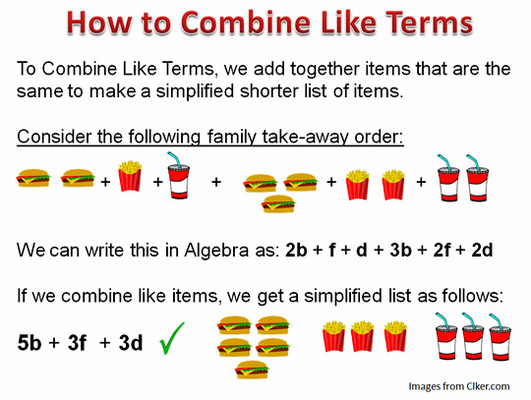Combining Like Terms
3 years ago
troche
Save
Edit
Host a game
Live GameLive
Homework
Solo Practice
Practice10 QuestionsShow answers
• Question 1
120 seconds
Q. Which of these are like terms in the following expression:
3x + 7 - 9x + 24y + 2x2
7, 24y
3x, 9x
3x, -9x
3x, -9x, 2x2
• Question 2
120 seconds
Q. Simplify by combining like terms:
5a + 2b - 3a + 4
8a + 2b + 4
2a + 2b + 4
8ab
4ab + 4
• Question 3
120 seconds
Q. Simplify by combining like terms:
4x2 - 3x + 11 -2x
4x2 - 5x + 11
11x + 11
16x2 - 5x + 11
10x2
• Question 4
120 seconds
Q. Which of these is the correct use of the distributive property for the following expression?
5(2x - 8)
10x - 8
10x + 40
7x - 3
10x - 40
• Question 5
120 seconds
Q. Simplify the following expression by combining like terms:
(3b - 4) - (4b + 6)
-b + 2
-b - 10
7b - 10
7b + 2
• Question 6
120 seconds
Q. Simplify the following expression by combining like terms:
3(2x - 4) + 6x - 1
12x - 13
12x - 5
11x - 2
-x
• Question 7
120 seconds
Q. Simplify the following expression by combining like terms:
x(3x + 4) + 2x
6x + 4
3x2 + 6x
3x2 + 2x + 4
9x2
• Question 8
120 seconds
Q. Factor the following expression:
5x + 15
3(x + 5)
5(x)
5(x + 3)
5x(15)
• Question 9
120 seconds
Q. Factor the following expression:
3a + 12b + 6b2
3b(a + 4 + 2b)
3(ab + b + 2b2)
b(13ab)
3(a + 4b + 2b2)
• Question 10
120 seconds
Q. Factor the following expression:
4x2 - 8x + 10xy